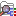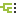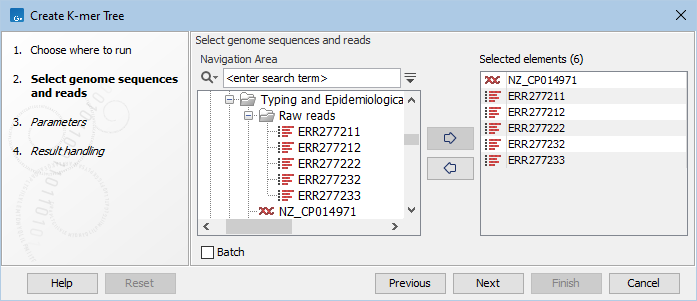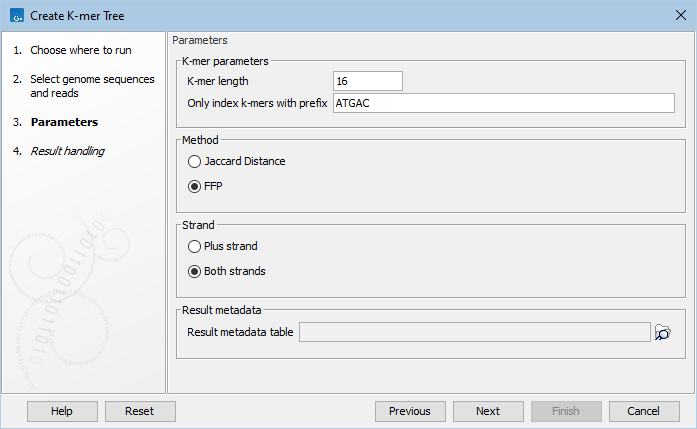Create K-mer Tree

The Create K-mer Tree tool may be helpful for identification of the closest common reference across samples. The tool uses reads, single sequences or sequence list as input and creates a distance-based phylogenetic tree. There are two ways to initiate creation of a k-mer tree: either from the Result Metadata Table (see the section on Running analysis directly from the Result Metadata Table), or from the Toolbox.

To run the Create K-mer Tree from the toolbox:

Typing and Epidemiology (beta) () | Create K-mer Tree ()

Input files can be specified step-by-step like shown in figure 14.15 or by selecting data recursively by right-clicking on the folder name and selecting Add folder contents (recursively). If using the recursive option, remember to double check that files relevant for the downstream analysis are selected.Figure 14.15: Selection of individual reads and single sequences or sequence list to be included in the K-mer tree analysis.

Specify the following parameters (figure 14.16):Figure 14.16: Various parameters may be set before generation of a K-mer tree.

The K-mer trees are constructed using a Neighbour Joining method, which makes use of a distance function, either Jaccard Distance or Feature Frequency Profile via Jensen-Shannon divergences (FFP). In both cases, the distance can assume values between 0 (exactly same k-mer distribution) and 1 (completely different k-mer distribution).

Branch lengths depend on the distance function used. Specifically, if one sums up all the branch length of all the branches connecting two leaves, one can get the distance between the two organisms the leaves represent.

Subsections